## 1. Introduction

In this tutorial, we’ll discuss the difference between various types of trees: Segment Tree, Interval Tree, Range Tree, and Binary Indexed Tree. It’s important to understand the structure and the usage of these tree types.

## 2. Preliminary Notes

The basic versions of the structures discussed in this article only work for static data. The element set is fixed, and we build the data structures on top of the fixed data.

Segment Tree and Binary Index Tree allow modification of existing elements. Nevertheless, their basic versions don’t support adding new elements or removing existing ones. Additionally, all structures have dynamic versions supporting element addition and removal. Those advanced augmented structures are out of our discussion scope.

## 3. Segment Tree

Segment Tree (ST) is a generic data structure that can be applied to solve range query problems.

### 3.1. Overview

Assume we have an 8-element array. Consider we want to solve the range sum query (RSQ) problem. When dealing with RSQ, we need to calculate sums on subarrays ofquickly. If we use the brute-force solution, RSQ takestime in the worst case, whereis the number of elements in the array.

ST is a binary tree defined in a hierarchical way: the root node contains the sum of the whole array, the left child node contains the sum of the left half, and the right child node contains the sum of the right half of the array. When the number of elements is a power of 2, ST becomes a complete binary tree. Otherwise, its form is asymmetric but doesn’t affect operations complexity.

ST allows us to perform RSQ in. Let’s build ST out offor solving the RSQ problem: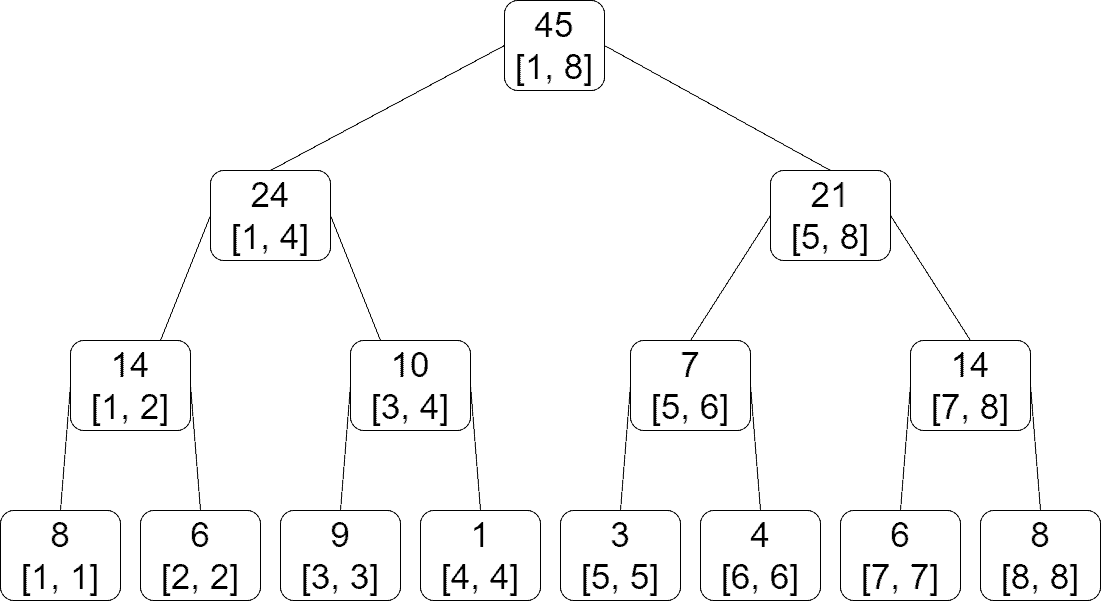A node contains the range for which it’s responsible and the sum of the elements on that range. For example, the root is responsible for the whole array; thus, its range contains all the elements. Contrarily, the leaves are single elements, and their ranges only contain themselves.

Similarly, ST for solving range minimum query (RMQ) takes the form: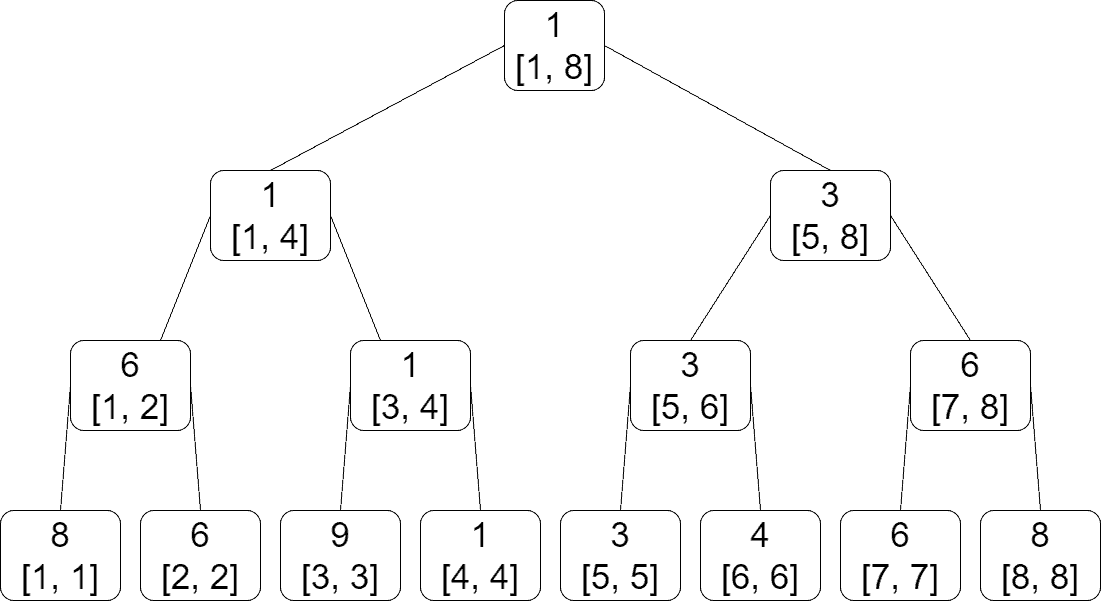The case of the range maximum query is similar, with the difference that we keep the maximum values in nodes instead of sums or minimums.

### 3.2. Use Cases

ST is used to solve a wide range of range query problems:

• RSQ
• RMQ (minimum or maximum)
• Count objects in a range with some property, e.g., the number of zero elements in a range
• Report objects in a range with some property, e.g., get all negative elements in a range

We’ve enumerated typical use cases for ST. Besides, there’re a lot of possible applications of ST that one can think of.

## 4. Interval Tree

An interval Tree (IT) is a computational geometry data structure. It enables us to quickly find out which intervals contain the given point or which intervals intersect with the given interval.

Initially, we have a set of intervals. For simplicity, we consider the 1-dimensional case and deal with intervals, but IT can be generalized to multidimensional applications. For example, when considering the 2-dimensional case, we can query rectangular regions and determine which rectangles intersect with the given one.

An IT is designed for cases when intervals are parallel to coordinate lines. For the 1-dimensional case, these simply are intervals with endpoints on theabscissa. Likewise, for the 2-dimensional case, we consider rectangles with sides parallel to coordinate lines.

### 4.1. Overview

Before building an IT, we put all the interval endpoints into an array and sort it. Note that we take into account repeating elements. Thus, if there’reintervals, we get an array withpoints and sort it in increasing order.

Next, we get the middle point and check which intervals contain the given point. The chosen point, along with all the intervals that contain that point, is added to the root node of IT. The left subtree will contain all the intervals to the left, and the right subtree will contain all the intervals to the right of the chosen point. The left and right subtrees are built similarly, using the appropriate parts of the sorted point array and the rest of the intervals.

For search efficiency, we don’t explicitly store intervals in nodes. Instead, we keep two sorted lists of left and right endpoints. Let’s sum all this up with an example.

Assume we have a 10-element set of intervals. We can see IT forbelow: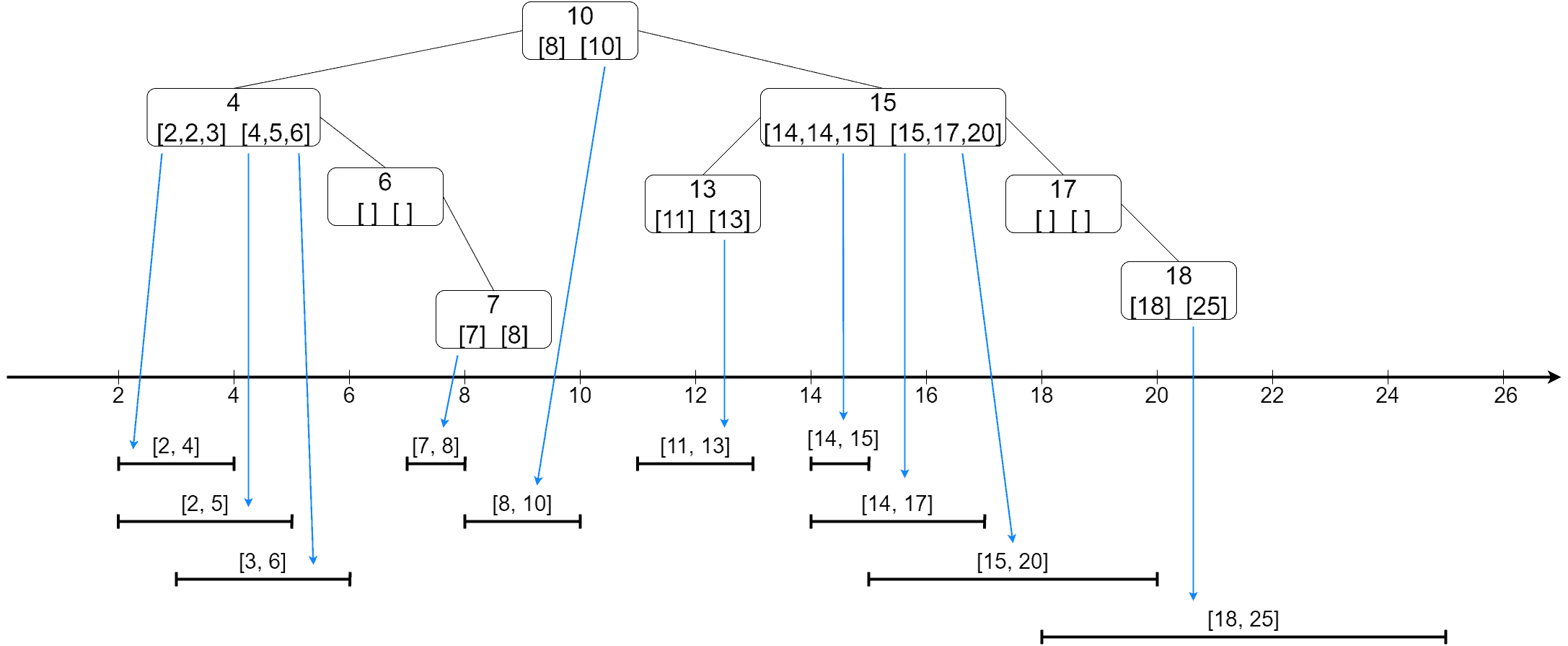Blue arrows point to the actual intervals that are contained in the node. For example, intervals, andcontain point 4, and their endpoints are listed in the appropriate lists of the root’s left child.

An IT is a balanced binary tree. It can answer counting queries (how many intervals contain the given point) intime. Additionally, it can answer fetching queries (which intervals contain the given point) intime, whereis the number of intervals to be returned by the query.

## 5. Range Tree

Range Tree (RT) is another computational geometry data structure. It solves the problem opposite to the one solved by IT: given a set of points, count or find all the points that the given interval contains.

### 5.1. Overview

As in the case of an IT, we consider our points are 1-dimensional, i.e., we simply have a set of numbers. Let’s fix the set.

An RT’s structure is very similar to a BST. A node contains a value, and all the numbers that are less are in the left, and all the numbers that are greater or equal are in the right subtree. Note that the values in the nodes may or may not be from. In our example, all the values in nodes are from. Besides a value, a node holds an interval that covers all the leaf elements of the tree rooted at the node.

The value in a node is a split point – it’s used to understand which direction the search should go. If the query interval contains the value, the search continues to both subtrees or terminates in the current node, depending on the query type and the intersection specifics with the node interval. Otherwise, the search either goes left or right. The interval in a node is used to decide whether there’s a sense to go in a direction. Also, it shows how the query interval intersects with the remaining search space. If the query interval doesn’t intersect with the interval in the node, then there’s no need to carry on the search.

Let’s construct an RT out of: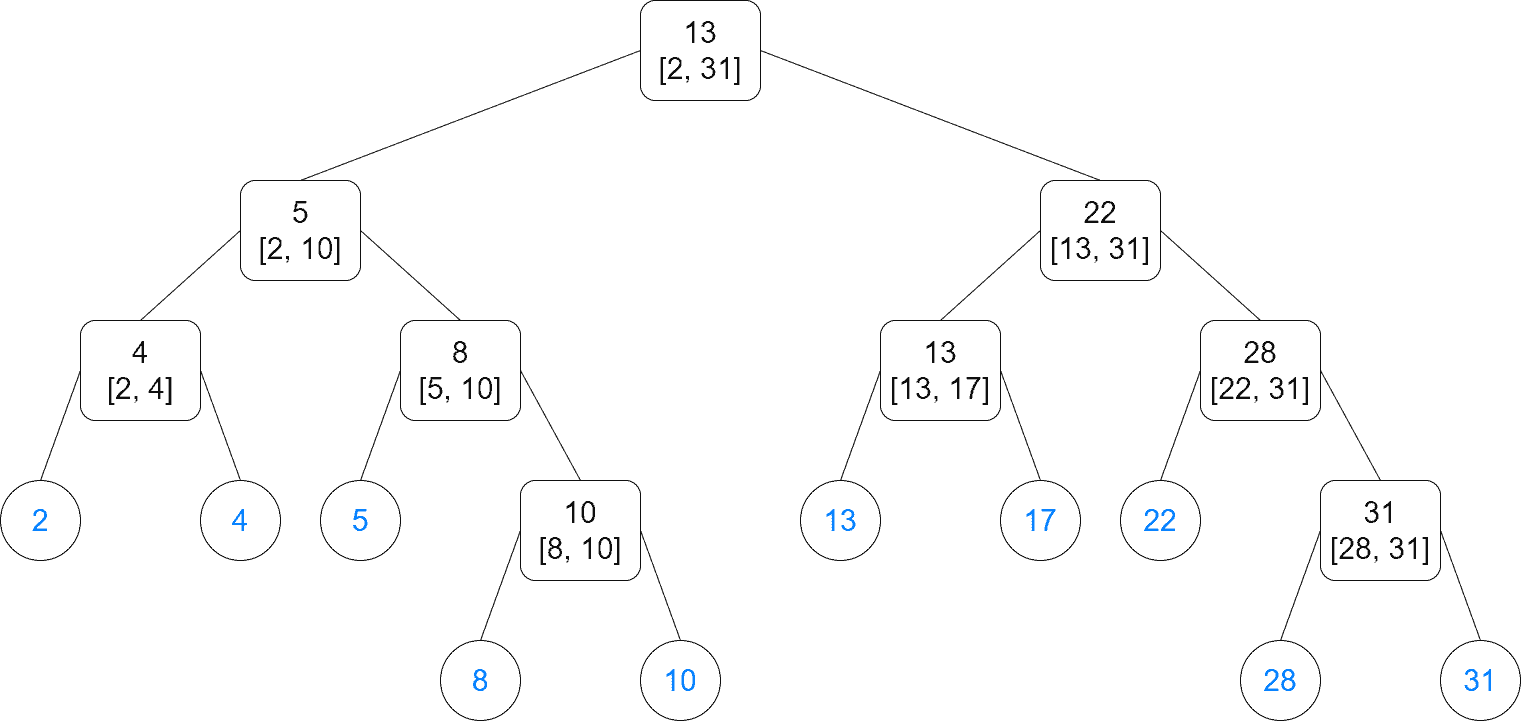The elements ofare tree leaves in blue. The rest are internal nodes that are only used for search. Note that the left children that are leaves can have values equal to the parent, while the left children that are internal nodes must strictly be less.

Similarly to IT, RT answers counting queries inand fetching queries in. An RT can be generalized to multidimensional cases, assuming the query region planes are parallel to coordinate planes.

## 6. Binary Indexed Tree

A Binary Index Tree (BIT), or Fenwick Tree, is a scheme to precalculate sums on an array. It allows answering RSQ intime. We don’t usually construct an explicit tree for a BIT. Instead, a BIT can be represented in an array having the same number of elements as the original array.

### 6.1. Overview

Suppose we have a 9-element 1-indexed array. The BIT is another arraydefined as follows:, where, is a function defining the start index for each. Thus,holds the sum of a subarray ending at.

We can find a visual representation of a BIT forbelow: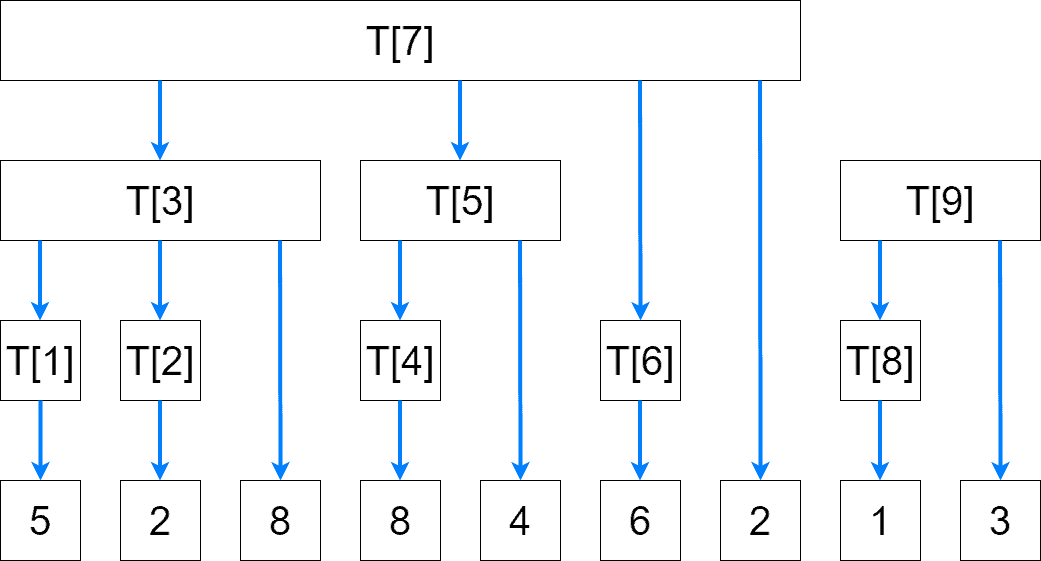Blue arrows indicate the dependencies for calculating values of. For example,, while.

## 7. How to Choose the Right Tree?

We can use an ST for most of the problems based on range queries. Additional augmentation might be needed to make an ST work for a specific application.

Both an ST and BIT can be used in RSQ problems, though a BIT is much easier to implement.

We use an IT to find intervals intersecting the given point or interval. Finally, an RT is used for finding points contained in the given interval.

## 8. Conclusion

In this tutorial, we’ve discussed the difference between Segment Tree, Binary Index Tree, Interval Tree, and Range Tree.

First, we’ve presented the idea of each tree. Then, we visualized their structure to have a better understanding of their internals. Finally, we’ve explained when to use them.